# NCERT Solutions for Understanding Elementary Shapes Chapter 5

In this page we have NCERT Solutions for Class 6 Maths Understanding Elementary Shapes Chapter 5 Ex 5.1,5.2,5.3,5.4,5.5,5.6,5.7,5.8,5.9. Hope you like them and do not forget to like , social share and comment at the end of the page.
Topic of 3-D figures is deleted from this chapter as per rationalized syllabus.So you may ignore the exercise 5.9
Table of Content

## Exercise 5.1

Question 1
What is the disadvantage in comparing line segments by mere observation?
You may be correct when the difference is obvious
But comparison become difficult when the difference in lengths between these two may not be obvious.

Question 2
Why is it better to use a divider than a ruler, while measuring the length of a line segment?
Ruler can be error prone because of two reasons
(a) thickness of the ruler
(b) Incorrect positioning of the eye will give wrong measurement
So, it is better to use divider than a ruler

Question 3.
Draw any line segment, say AB. Take any point C lying in between A and B.
Measure the lengths of AB, BC and AC. Is AB = AC + CB?
[Note: If A, B, C are any three points on a line such that AC + CB = AB, then we can be sure that C lies between A and B.]
Draw the line and then measure each line segment to check it

Question 4.
If A, B, C are three points on a line such that AB = 5 cm, BC = 3 cm and AC = 8 cm, which one of them lies between the other two?
Given that,
AB =5 cm
BC = 3 cm
AC = 8 cm
Here, AC = AB + BC
Therefore, point B is lying between A and C.

Question 5.
Verify, whether D is the midpoint of AG.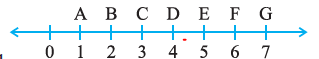We have
DG= 3 units
AG =6 units
So, D is the midpoint of AG

Question 6.
If B is the mid-point of AC and C is the mid-point of BD, where A, B, C, D lie on a straight line, say why AB = CD?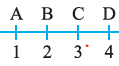AB =BC (as B is mid-point)
BC=CD (as C is the mid-point)
Therefore
AB=CD

Question 7.
Draw five triangles and measure their sides. Check in each case, if the sum of the lengths of any two sides is always less than the third side.

## Exercise 5.2

Question 1
What fraction of a clockwise revolution does the hour hand of a clock turn through, when it goes from
(a) 3 to 9
(b) 4 to 7
(c) 7 to 10
(d) 12 to 9
(e) 1 to 10
(f) 6 to 3

(a) 1/2 or two right angles.
(b) 1/4 or one right angle.
(c) 1/4 or one right angle.
(d) 3/4 or three right angles.
(e) 3/4 or three right angles.
(f) 3/4 or three right angles.

Question 2. Where will the hand of a clock stop if it?
(a) starts at 12 and makes ½ of a revolution, clockwise?
(b) starts at 2 and makes ½ of a revolution, clockwise?
(c) starts at 5 and makes ¼ of a revolution, clockwise?
(d) starts at 5 and makes ¾ of a revolution, clockwise?
(a) At 6
(b) At 8
(c) At 8
(d) At 2

Question 3
Which direction will you face if you start facing
(a) east and make ½ of a revolution clockwise?
(b) east and make $1 \frac {1}{2}$ of a revolution clockwise?
(c) west and make ¾ of a revolution anti-clockwise?
(d) south and make one full revolution?
(Should we specify clockwise or anti-clockwise for this last question? Why not?)
a) west
b) west
c) North
d) south

Question 4
What part of a revolution have you turned through if you stand facing
(a) east and turn clockwise to face north?
(b) south and turn clockwise to face east?
(c) west and turn clockwise to face east?
a) ¾
b) ¾
c) ½

Question 5.
Find the number of right angles turned through by the hour hand of a clock when it goes from
(a) 3 to 6
(b) 2 to 8
(c) 5 to 11
(d) 10 to 1
(e) 12 to 9
(f) 12 to 6
(a) One right angle
(b) Two right angles
(c) Two right angles
(d) One right angle
(e) Three right angles
(f) Two right angles

Question 6.
How many right angles do you make if you start facing
(a) south and turn clockwise to west?
(b) north and turn anti-clockwise to east?
(c) west and turn to west?
(d) south and turn to north?
(a) One right angle
(b) Three right angles
(c) Four right angles
(d) Two right angles

Question 7
Where will the hour hand of a clock stop if it starts
(a) from 6 and turns through 1 right angle?
(b) from 8 and turns through 2 right angles?
(c) from 10 and turns through 3 right angles?
(d) from 7 and turns through 2 straight angles?
(a) At 9
(b) At 2
(c) At 7
(d) At 7

## Exercise 5.3

Question 1
Match the correct options
 (A) Straight angle (i) Less than one-fourth of a revolution (B) Right angle (ii) More than half a revolution (C) Acute angle (iii) Half of a revolution (D) Obtuse angle (iv) One-fourth of a revolution (E) Reflex angle (v) Between 14 and 12 of a revolution (vi) One complete revolution

(A) Straight angle — iii) Half of a revolution
As $180^0$ is considered as a straight line and half of revolution is also $180^0$
(B) Right angle — iv) One-fourth of a revolution
As Right angle and One-fourth of a revolution are both $90^0$
(C) Acute angle — i) Less than one-fourth of a revolution
As The angle which is less than 90 is called acute angle and less than one-fourth of a revolution is the angle less than $90^0$
(D) Obtuse angle — v) Between 14 and 12 of a revolution
Obtuse angle   is the angle which is greater than 90 and less than 180 and the value between 14 and 12 of a revolution also lies between 90 and 180
(E) Reflex angle – ii) More than half a revolution
Reflex angle is the angle which is greater than $180^0$ but less than $360^0$ and more than half a revolution is the angle whose measure is greater than $180^0$

Question 2
Classify each one of the following angles as right, straight, acute, obtuse or reflex: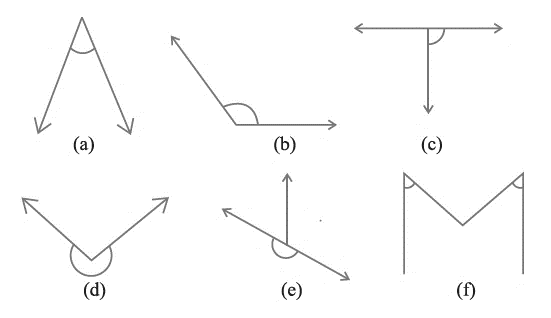(a) Acute
(b) obtuse
(c) Right angle
(d) reflex angle
(e) straight angle
(f) Acute

## Exercise 5.4

Question 1.
What is the measure of
(i) a right angle?
(ii) a straight angle?
(a) $90^0$
(b) $180^0$

Question 2.
Say True or False:
(a) The measure of an acute angle < 90°.
(b) The measure of an obtuse angle < 90°.
(c) The measure of a reflex angle > 180°.
(d) The measure of one complete revolution = 360°.
(e) If m∠ÐA = 53° and m∠ÐB = 35°, then m∠ÐA > Ðm∠B.
(a) true
(b) false
(c) true
(d) true
(e) True

Question 3.
Write down the measures of
(a) some acute angles.
(b) some obtuse angles.
(give at least two examples of each).
(i) Acute angles
$60^0$
$45^0$
(ii) Obtuse angles
$120^0$
$160^0$
Question 4.
Measure the angles given below using the Protractor and write down the measure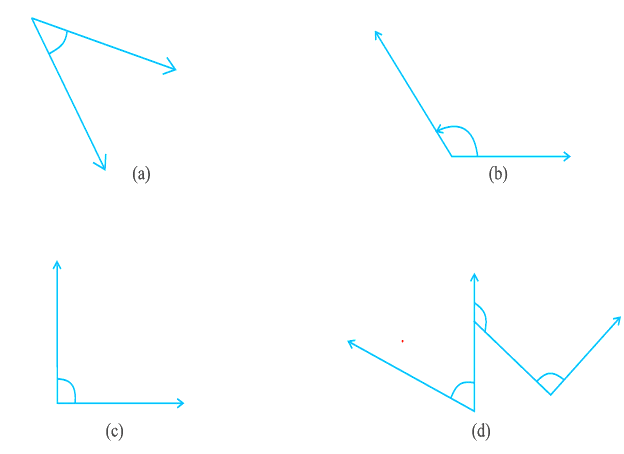a) $40^0$
b) $130^0$
c) $90^0$
d) $60^0$

Question 5
Which angle has a large measure?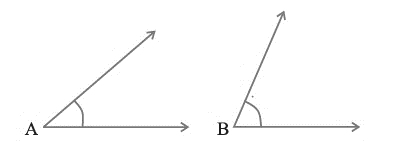First estimate and then measure.
Measure of Angle A =
Measure of Angle B =
A measure $40^0$
B measures $60^0$
∠A has the largest angle.

Question 6
From these two angles which has larger measure? Estimate and then confirm by measuring them.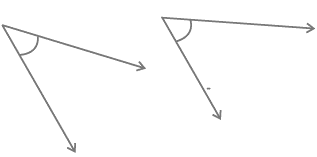The angles are $70^0$ and $75^0$

Question 7
Fill in the blanks with acute, obtuse, right or straight:
(a) An angle whose measure is less than that of a right angle is______.
(b) An angle whose measure is greater than that of a right angle is ______.
(c) An angle whose measure is the sum of the measures of two right angles is _____.
(d) When the sum of the measures of two angles is that of a right angle, then each one of them is ______.
(e) When the sum of the measures of two angles is that of a straight angle and if one of them is acute then the other should be _______.
(a) acute
(b) obtuse (if the angle is less than 180°)
(c) straight
(d) acute
(e) an obtuse angle.

Question 8
Find the measure of the angle shown in each figure. (First estimate with your eyes and then find the actual measure with a protractor).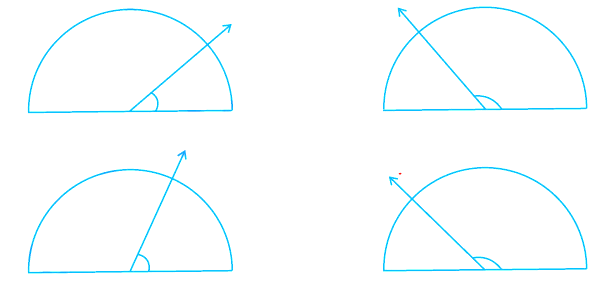The angle measures
(i)$30^0$
(ii)$120^0$
(iii)$60^0$
(iv)$180^0$

Question 9
Find the angle measure between the hands of the clock in each figure: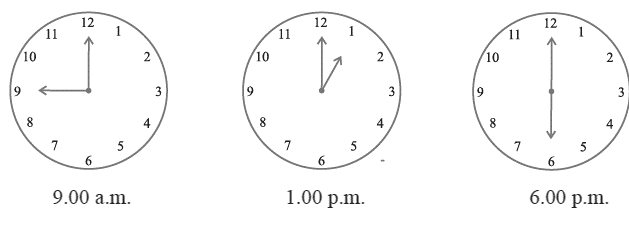90°, 30°, 180°

Question 10
Investigate
In the given figure, the angle measures 30°. Look at the same figure through a magnifying glass.
Does the angle become larger? Does the size of the angle change?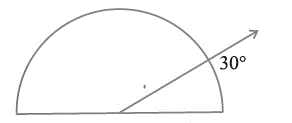The angle does not change

Question 11
Measure and classify each angle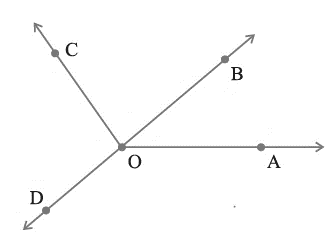Angle Measure Type ∠AOB – – ∠AOC – – ∠BOC – – ∠DOC – – ∠DOA – – ∠DOB – –

 Angle Measure Type ∠AOB 50 Acute ∠AOC 130 Obtuse ∠BOC 80 Acute ∠DOC 100 Obtuse ∠DOA 145 Obtuse ∠DOB 180 Straight

## Exercise 5.5

Question 1.
Which of the following are models for perpendicular lines?
(a) The adjacent edges of a table top.
(b) The lines of a railway track.
(c) The line segments forming the letter ‘L’.
(d) The letter V.
(a)Perpendicular
(b) Not perpendicular
(c) Perpendicular
(d) Not perpendicular

Question 2.
Let PQ be the perpendicular to the line segment XY. Let PQ and XY intersect in the point A. What is the measure of ∠PAY?
90

Question 3
There are two set-squares in your box. What are the measures of the angles that are formed at their corners? Do they have any angle measure that is common?
One is a 30° – 60° – 90° set square; the other is a 45° – 45° – 90° set square.
The angle of measure 90° (i.e. a right angle) is common between them.

Question 4.
Study the diagram. The line l is perpendicular to line m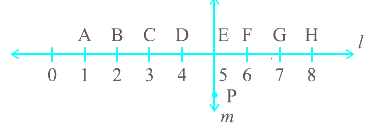(a) Is CE = EG?
(b) Does PE bisect CG?
(c) Identify any two-line segments for which PE is the perpendicular bisector.
(d) Are these true?
(i) AC > FG
(ii) CD = GH
(iii) BC < EH.
(a) Yes, as same units
(b) Yes, as CE =EG
(c) BH, DF
(d) All are true.
(i)True, (ii) True, (iii) True

## Exercise 5.6

Question 1
Name the types of following triangles:
(a) Triangle with lengths of sides 7 cm, 8 cm and 9 cm.
(b) $\Delta ABC$ with AB = 8.7 cm, AC = 7 cm and BC = 6 cm.
(c) $\Delta PQR$ such that PQ = QR = PR = 5 cm.
(d) $\Delta DEF$ with m∠d= 90°
(e) $\Delta XYZ$ with m∠Y= 90° and XY = YZ.
(f) $\Delta LMN$ with m∠L = 30°, m∠M = 70° and m∠N= 80°.
(a) Scalene triangle
(b) Scalene triangle
(c) Equilateral triangle
(d) Right triangle
(e) Isosceles right triangle
(f) Acute-angled triangle

Question 2:
Match the following-:
Measures of Triangle                   Type of Triangle
(i)3 sides of equal length             (a) Scalene
(ii) 2 sides of equal length               (b) Isosceles right angled
(iii) All sides are of different length (c) Obtuse angled
(iv) 3 acute angles                           (d) Right angled
(v) 1 right angle                              (e) Equilateral
(vi) 1 obtuse angle                           (f) Acute angled
(vii) 1 right angle with two sides of equal length (g) Isosceles
(i)3 sides of equal length                (e) Equilateral
(ii) 2 sides of equal length               (g) Isosceles
(iii) All sides are of different length   (a) Scalene
(iv) 3 acute angles                           (f) Acute angled
(v) 1 right angle                               (d) Right angled
(vi) 1 obtuse angle                           (c) Obtuse angled
(vii) 1 right angle with two sides of equal length   (b) Isosceles right angled

Question 3
Name each of the following triangles in two different ways: (you may judge the nature of the angle by observation)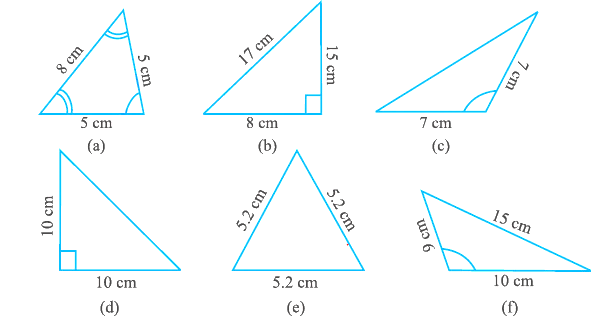(a) Acute-angled and isosceles.
(b) Right-angled and scalene.
(c) Obtuse-angled and isosceles.
(d) Right-angled and isosceles.
(e) Equilateral and acute angled.
(f) Obtuse-angled and scalene.

Question 4
Try to construct triangles using match sticks. Some are shown here.
Can you make a triangle with
(a) 3 matchsticks?
(b) 4 matchsticks?
(c) 5 matchsticks?
(d) 6 matchsticks?
(Remember you must use all the available matchsticks in each case)
Name the type of triangle in each case.
If you cannot make a triangle, think of reasons for it.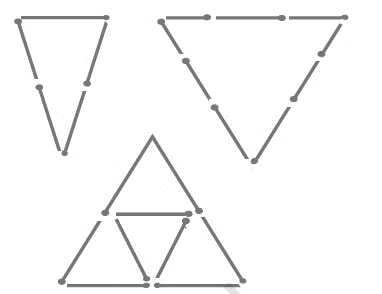(a) Possible (equilateral triangle)
(b) not possible. We cannot build the triangle, as then sum of two sides would be equal to another side). Square is possible
(c) possible. Isosceles triangle (2-2-1 sticks)
(d) possible. Equilateral triangle (2-2-3 sticks)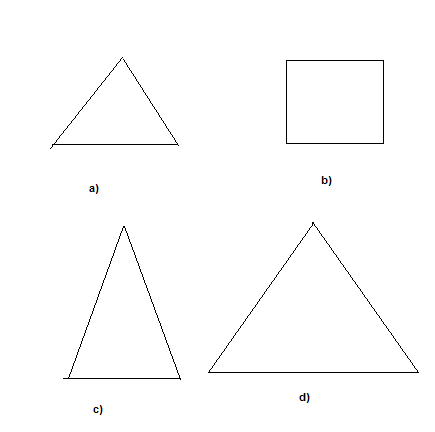## Exercise 5.7

Question 1
Say True or False:
(a) Each angle of a rectangle is a right angle.
(b) The opposite sides of a rectangle are equal in length.
(c) The diagonals of a square are perpendicular to one another.
(d) All the sides of a rhombus are of equal length.
(e) All the sides of a parallelogram are of equal length.
(f) The opposite sides of a trapezium are parallel.
(a) True
(b) True
(c) True
(d) True
(e) False as opposite sides are equal only
(f) False. Both the opposite sides are not parallel

Question 2.
Give reasons for the following:
(a) A square can be thought of as a special rectangle.
(b) A rectangle can be thought of as a special parallelogram.
(c) A square can be thought of as a special rhombus.
(d) Squares, rectangles, parallelograms are all quadrilaterals.
(e) Square is also a parallelogram.
(i) A square has all the properties of a rectangle as its opposite sides are equal also all the angles are right angled.
(ii) A rectangle has also its opposite sides equal as well as parallel. So, it has all the properties of the parallelogram,
(iii) In a rhombus all the sides are equal also diagonals are perpendicular to each other. And square have the same property.
(iv) All these are four-sided polygons made of line segments.
(v) A square also has its opposite sides equal as well as parallel.

Question 3.
A figure is said to be regular if its sides are equal in length and angles are equal in measure. Can you identify the regular quadrilateral?
A square is a regular quadrilateral.

## Exercise 5.8

Question 1
Examine whether the following are polygons. If anyone among them is not, say why?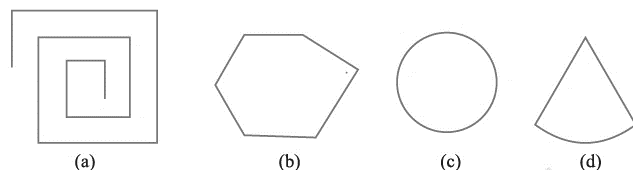(a) As the figure is not closed, therefore, the shape is not a polygon.
(b) As it is closed by line segments hence it is a polygon.
(c) As it is not made of line of segments it is not a polygon.
(d)As it has curved surface and is made up of line of segments.it is not a polygon

Question 2
Name each polygon.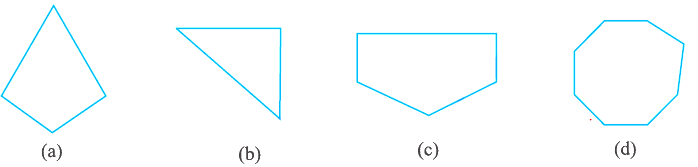(b) Triangle
(c) Pentagon
(d) Octagon

Question 3
Draw a rough sketch of a regular hexagon. Connecting any three of its vertices, draw a triangle. Identify the type of the triangle you have drawn.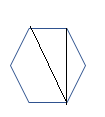The triangle drawn is right angle triangle

Question 4.
Draw a rough sketch of a regular octagon. (Use squared paper if you wish). Draw a rectangle by joining exactly four of the vertices of the octagon.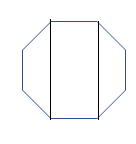Question 5.
A diagonal is a line segment that joins any two vertices of the polygon and is not a side of the polygon. Draw a rough sketch of a pentagon and draw its diagonals.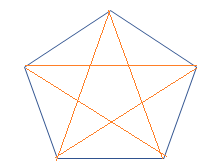## Exercise 5.9

Question 1
Match the column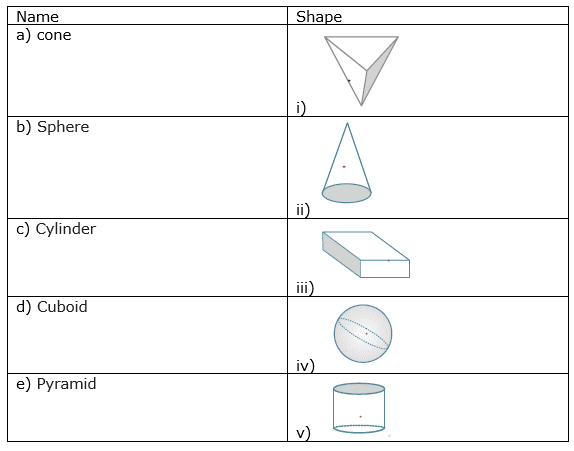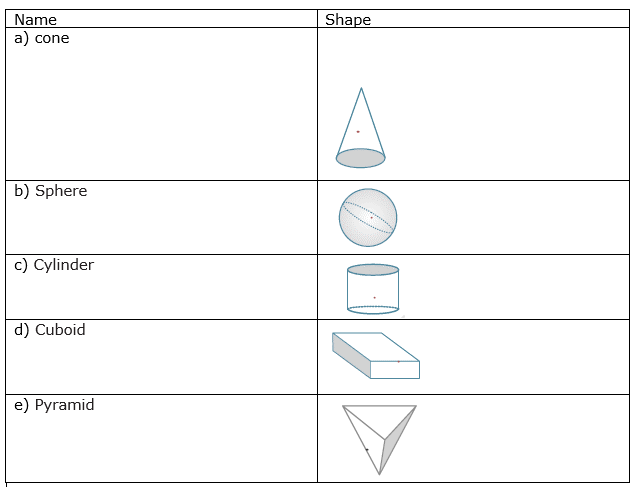Question 2
What shape is
(b) A brick?
(c) A match boxes?

(a) Cuboid
(b) Cuboid
(c) Cuboid
(d) Cylinder
(e) Sphere

## Try these NCERT work book solutions

1. What is the angle name for half a revolution?
2 right angles

2. What is the angle name for one-fourth revolution?
1 right angle

3.
The hour hand of a clock moves from 12 to 5. Is the revolution of the hour hand more than 1 right angle?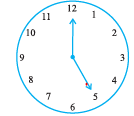True
4. What does the angle made by the hour hand of the clock look like when it moves from 5 to 7. Is the angle moved more than 1 right angle?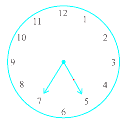60 degree
Less than 90 degree

5. Draw the following and check the angle with your RA tester.
(a) going from 12 to 2
(b) from 6 to 7
(c) from 4 to 8
(d) from 2 to 5
a.60 degree
b. 30 degree
c. 120 degree
d. 90 degree

6.
a. If all the angles in a triangle are equal, then its sides are also __________
b. If all the sides in a triangle are equal, then its angles are __________
c. If two sides of a triangle are equal, it has ________ equal angles.
and if two angles of a triangle are equal, it has  _________ equal sides.
d. If none of the angles of a triangle are equal then none of the sides are ___________
e. If the three sides of a triangle are unequal then, the three angles are also __________
a. Equal
b. Equal
c. two,two
d. equal
e. unequal

7.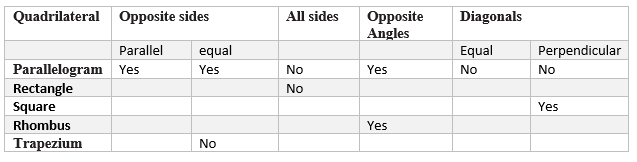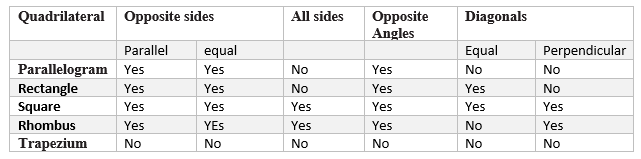8.
A cuboid looks like a rectangular box.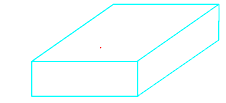It has ___ faces. Each face has ____ edges.
Each face has ____ corners (called vertices).

b. A cube is a cuboid whose edges are all of equal length.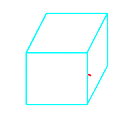It has ______ faces.
Each face has ____ edges.
Each face has ____ corners (called vertices).

c. A triangular pyramid has a triangle as its base. It is also known as a tetrahedron.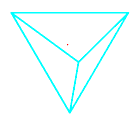Faces : _______
Edges : _______
Corners : _______

d. A square pyramid has a square as its base.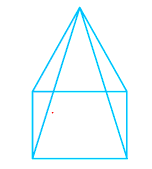Faces : _______
Edges : _______
Corners : _______

5. A triangular prism looks like the shape of a Kaleidoscope. It has triangles as its bases.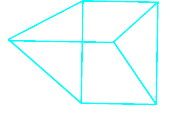Faces : _______
Edges : _______
Corners : _______
a. 6,4,4
b. 6,4,4
c. 4,6,4
d. 5,8,5
e. 5,9,6

• NCERT Solutions### Practice Question

Question 1 What is $\frac {1}{2} + \frac {3}{4}$ ?
A)$\frac {5}{4}$
B)$\frac {1}{4}$
C)$1$
D)$\frac {4}{5}$
Question 2 Pinhole camera produces an ?
A)An erect and small image
B)an Inverted and small image
C)An inverted and enlarged image
D)None of the above